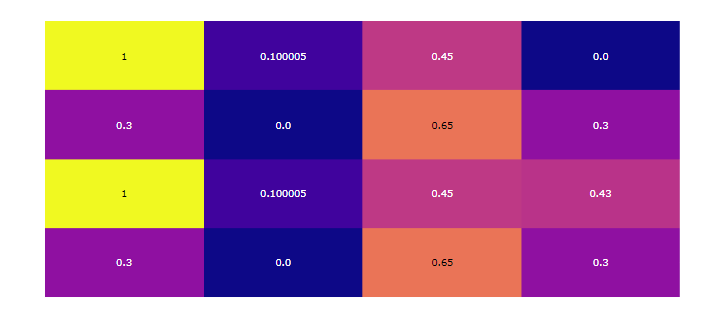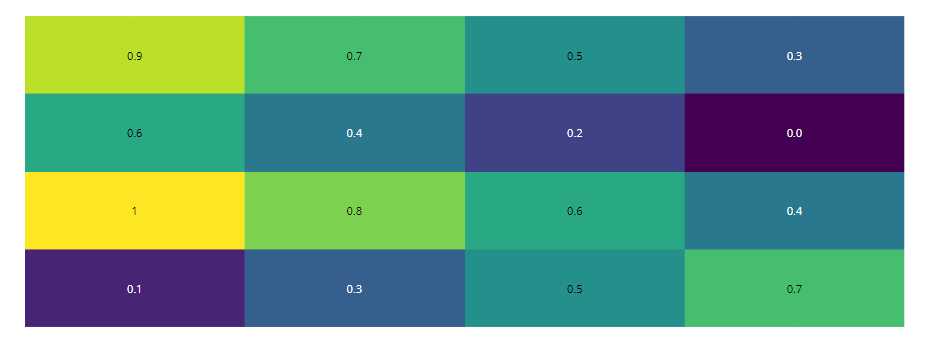Open In App

# plotly.figure_factory.create_annotated_heatmap() function in Python

Plotly library of Python can be very useful for data visualization and understanding the data simply and easily.

## plotly.figure_factory.create_annotated_heatmap

Function that creates annotated heatmaps and adds annotations to each cell of the heatmap.

Syntax:plotly.figure_factory.create_annotated_heatmap(z, x=None, y=None, annotation_text=None, colorscale=’Plasma’, font_colors=None, showscale=False, reversescale=False, **kwargs)

Parameter:

z ((list[list]|ndarray)) – it describe the z matrix to create heatmap.

x ((list)) – it describe the x axis labels.

y ((list)) – it describe the y axis labels.annotation_text ((list[list]|ndarray)) – Text strings for annotations. Should have the same dimensions as the z matrix. If no text is added, the values of the z matrix are annotated. Default = z matrix values.

colorscale ((list|str)) – it describe heatmap colorscale.

font_colors ((list)) – it describes the list of two color strings: [min_text_color, max_text_color]

showscale ((bool)) – it display colorscale with Default as False

reversescale ((bool)) – it reverse colorscale with Default as False

Example 1: Simple annotated heatmap with default configuration

## Python3

 `import` `plotly.figure_factory as ff`` ` ` ` `z ``=` `[[``0.300000``, ``0.00000``, ``0.65``, ``0.300000``],``     ``[``1``, ``0.100005``, ``0.45``, ``0.4300``],``     ``[``0.300000``, ``0.00000``, ``0.65``, ``0.300000``],``     ``[``1``, ``0.100005``, ``0.45``, ``0.00000``]]``fig ``=` `ff.create_annotated_heatmap(z)``fig.show()`

Output:Example 2: Defined colorscale

## Python3

 `import` `plotly.figure_factory as ff`` ` `z ``=` `[[.``1``, .``3``, .``5``, .``7``],``     ``[``1``, .``8``, .``6``, .``4``],``     ``[.``6``, .``4``, .``2``, .``0``],``     ``[.``9``, .``7``, .``5``, .``3``]]`` ` `fig ``=` `ff.create_annotated_heatmap(z, colorscale``=``'Viridis'``)``fig.show()`

Output: